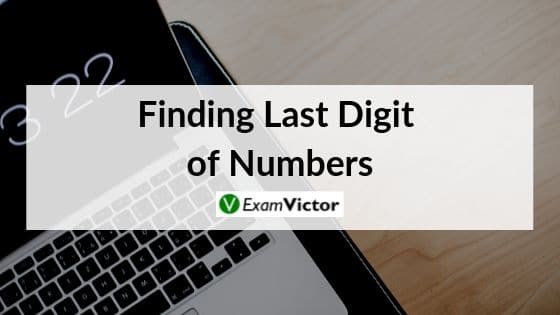# Finding Last Digit of NumbersLast Updated on Oct 15, 2020

Finding last digit of numbers in an interesting topic. Understanding this topic well is necessary to solve questions that are asked in various competitive exams in the Quant section – applicable to CAT, XAT, MAT, SNAP, IIFT, CLAT, AILET, DU LLB, any other entrance exam as well.

Recognizing patterns is a common way to attack these type of questions is to list out the initial expansions of a power to determine a pattern. Questions which ask about the last decimal digit of a power can be solved completely after proving that the pattern in question holds.

##### Shortcut Tricks to find last digit of any number

One Golden Rule: The fifth power of any number has the same units place digit as the number itself.

We are finding last digit(unit’s place) of x^y (x raised to the power of y).

The question will fit into one of the 4 cases mentioned below.

Case 1: When y is a multiple of 4 which is (4,8,12,16….) and x is a even number(2,4,6…. except even numbers ending with zero)

Format is (even^4n)

Then the last digit(unit’s place) of x^y=6

Example: 22^4=234256

16^8=4294967296

8^8=16777216

Numbers with zero at the end raised to any power will create zero in the unit’s place.

Case 2When y is a multiple of 4 which is (4,8,12,16…) and x is a odd number(1,3,7,9….except odd number with last digit as 5)

Format is (odd^4n)

Then the last digit(unit’s place) of x^y=1

Example: 3^12=531441

7^8=5764801

Case 3The last digit of anything to the power of x having unit place as 5 is 5.

Format is (number ending in 5^any natural number)

(any number ending in 5)^(any natural number)=number’s last digit is 5.

Example: 5^6=15625

Case 4 :In cases where the y is not a multiple of 4 hence like 4n+1,4n+2 or 4n+3 .

You just have to multiply the last digit once twice & thrice with itself starting with 1 in case of x as odd number and 6 in case of x as even number.

Example 1: 57^30

57 is odd number and 30 is 4n+2

57^28 is fitting in case 2 hence the last digit must be 1

Now that one is multiplied by 7 twice(1 x 7 x 7=49) to get to 57^30

Last digit is 9.

Example 2: 34^35

34 is even number and 35 is 4n+3

34^32 is fitting in case 1 hence the last digit must be 6

Now that 6 is multiplied by 4 thrice(6 x 4 x 4 x 4) =ends in 4 to get to 34^35.

Last digit is 6

To summarize the 4 cases.

1. Even number raised to 4n=6
2. Odd number raised to 4n=1
3. Numbers ending in 5 raised to any natural number=5
4. Numbers raised to 4n+1/2/3 should be multiplied with itself once or twice or thrice with 6 in case of even x and 1 in case of odd x.

Note: Numbers ending with 0,1,5,6 as last digit raised to any power will have same numbers repeated.

In case 1,I have excluded even numbers ending in zero(10,20,30,40….)

In case 2,I have excluded odd numbers ending in 5(5,15,25,35,45,55….)

Concept of Cyclicity is also discussed in the video.

Vivek Sir will explain tips and tricks to find last digit of a number in the video below. Check it out!